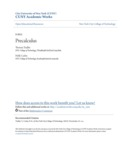## Open TextbooksPrecalculus

#### Contributors

Thomas Tradler - Author / Holly Carley - Author

#### Description

These are notes for a course in precalculus/ as it is taught at New York City College of Technology - CUNY (where it is offered under the course number MAT 1375). Our approach is calculator based. For this/ we will use the currently standard TI-84 calculator/ and in particular/ many of the examples will be explained and solved with it. However/ we want to point out that there are also many other calculators that are suitable for the purpose of this course and many of these alternatives have similar functionalities as the calculator that we have chosen to use. An introduction to the TI-84 calculator together with the most common applications needed for this course is provided in appendix A. In the future we may expand on this by providing introductions to other calculators or computer algebra systems. This course in precalculus has the overarching theme of “functions.” This means that many of the often more algebraic topics studied in the previous courses are revisited under this new function theoretic point of view. However/ in order to keep this text as self contained as possible we always recall all results that are necessary to follow the core of the course even if we assume that the student has familiarity with the formula or topic at hand. After a first introduction to the abstract notion of a function/ we study polynomials/ rational functions/ exponential functions/ logarithmic functions/ and trigonometric functions with the function viewpoint. Throughout/ we will always place particular importance to the corresponding graph of the discussed function which will be analyzed with the help of the TI-84 calculator as mentioned above. These are in fact the topics of the first four (of the five) parts of this precalculus course. In the fifth and last part of the book/ we deviate from the above theme and collect more algebraically oriented topics that will be needed in calculus or other advanced mathematics courses or even other science courses. This part includes a discussion of the algebra of complex numbers (in particular complex numbers in polar form)/ the 2-dimensional real vector space R 2 sequences and series with focus on the arithmetic and geometric series (which are again examples of functions/ though this is not emphasized)/ and finally the generalized binomial theorem.

#### Subject 1

Mathematics - Pure

#### Subject 2

Mathematics - Pure

#### Resources

Open Textbook Library